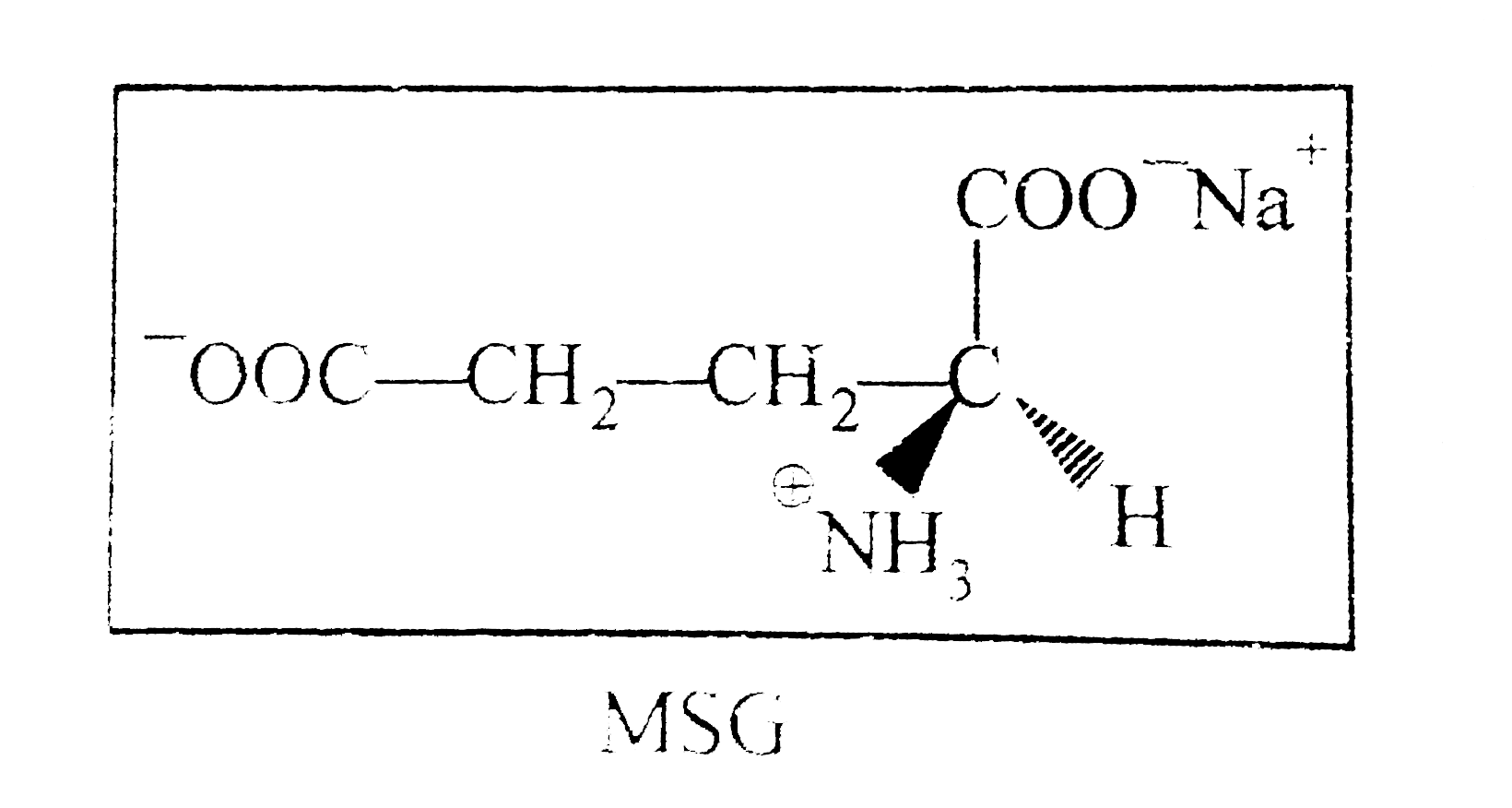# S(+) Mono Sodium Glutamate (MSG) is a flavour enhancer used in many foods. Fast foods often contain substantial amount of MSG and is widely used in Ch

227 views

closed
S(+) Mono Sodium Glutamate (MSG) is a flavour enhancer used in many foods. Fast foods often contain substantial amount of MSG and is widely used in Chines food. If one mole of above MSG was placed in 845 ml solution and passed through 200 mm tube, the observed rotation was found to be +9.6^(@).If 33.8g of (+) MSG was put in 338 ml solution and was mixed with 16.9 g of (-) MSG put in 169 ml solution and the final solution was passed through 400 mm tube. find out observed rotation of these final solution.
A. +1.6^(@)
B. +4.8^(@)
C. +3.2^(@)
D. none of these

by (65.9k points)
selected

[(+) MSG rArr  33.8 gm in 338 ml
(-) MSG rArr 16.9 gm in 169 ml
Optical purity in mixture (+) MSG =16.9 gm in 507 ml solution
C=16.9 /507 gm//ml " " l=4 dm
theta_("Observed")=[theta^(@)]xxC.l. " " =24xx16.9/507 xx4=+3.2^(@)]